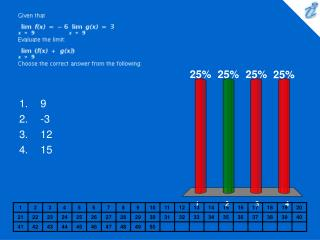DownloadDownload PresentationGiven that {image} {image} Evaluate the limit: {image} Choose the correct answer from the following:

# Given that {image} {image} Evaluate the limit: {image} Choose the correct answer from the following:

Download Presentation## Given that {image} {image} Evaluate the limit: {image} Choose the correct answer from the following:

- - - - - - - - - - - - - - - - - - - - - - - - - - - E N D - - - - - - - - - - - - - - - - - - - - - - - - - - -
##### Presentation Transcript

1. Given that {image} {image} Evaluate the limit: {image} Choose the correct answer from the following: • 9 • -3 • 12 • 15

2. 1. 2. 3. Evaluate the limit: {image} Choose the correct answer from the following: • {image} • {image} • {image}

3. 1. 2. 3. 4. If {image} for all x find: {image} Choose the correct answer from the following: • 1 • The limit does not exist • {image} • 7

4. Evaluate the limit: {image} Choose the correct answer from the following: • 0 • The limit does not exist • 8 • 1